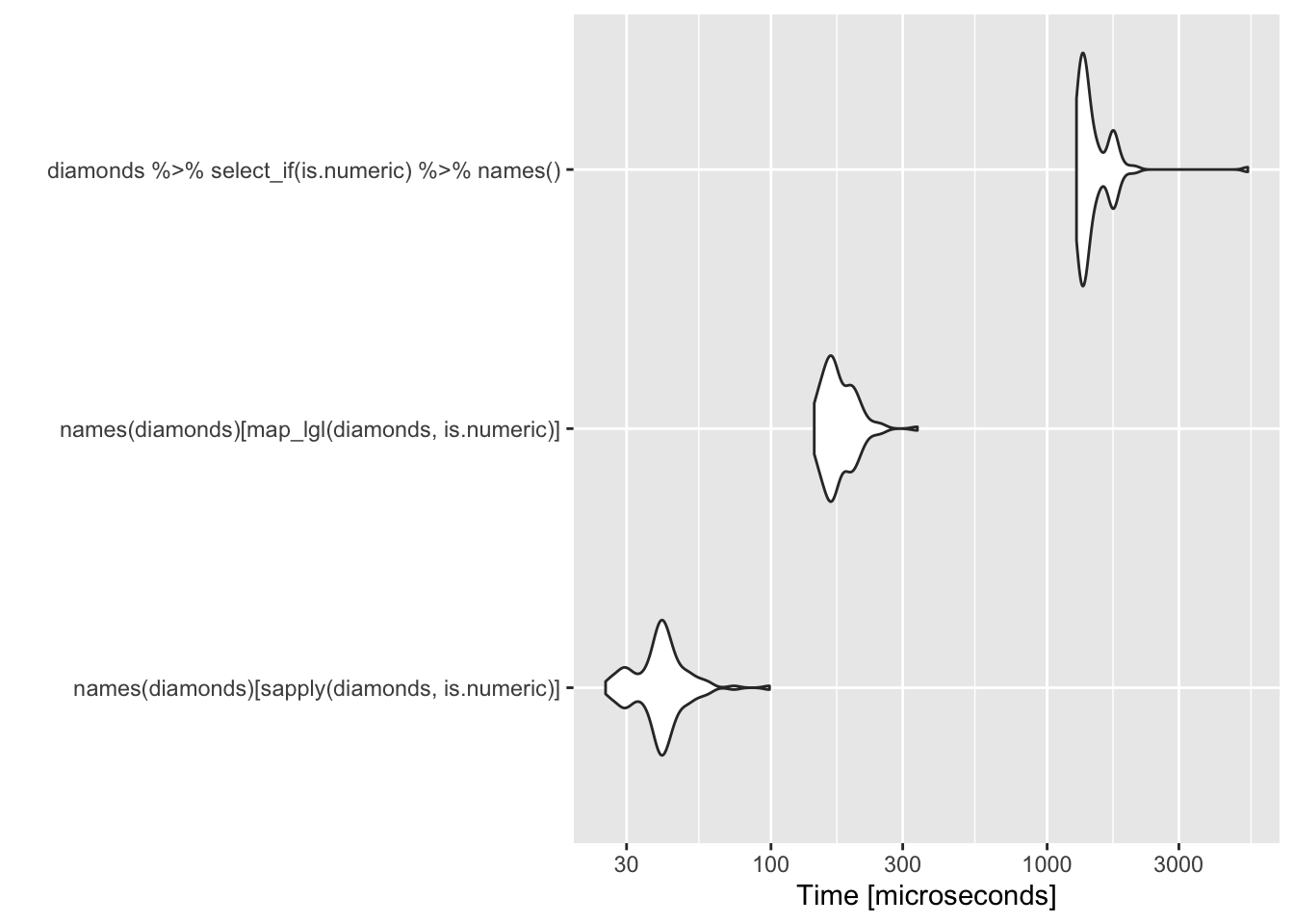# Selecting columns based on type

The tidyverse and, in particular, dplyr, provides functions to select columns from a data frame. There are three scoped functions available: select_all, select_if and select_at. In this post, we’ll look at a particular application of select_if, i.e., capturing the names of numeric variables.

A quick search using Google finds a few solutions to this problem. As an example data set, I’ll use the diamonds data set from the ggplot2 package.

names(diamonds)[sapply(diamonds, is.numeric)]
##  "carat" "depth" "table" "price" "x"     "y"     "z"

or, equivalently

names(diamonds)[map_lgl(diamonds, is.numeric)]
##  "carat" "depth" "table" "price" "x"     "y"     "z"

However, there is an elegant (to me) pipeline based solution using select_if.

diamonds %>% select_if(is.numeric) %>% names()
##  "carat" "depth" "table" "price" "x"     "y"     "z"

However, the elegance is at the expense of some efficiency.

library(microbenchmark)
microbenchmark(names(diamonds)[sapply(diamonds, is.numeric)],
names(diamonds)[map_lgl(diamonds, is.numeric)],
diamonds %>% select_if(is.numeric) %>% names()) %>%
autoplot()
## Coordinate system already present. Adding new coordinate system, which will replace the existing one.